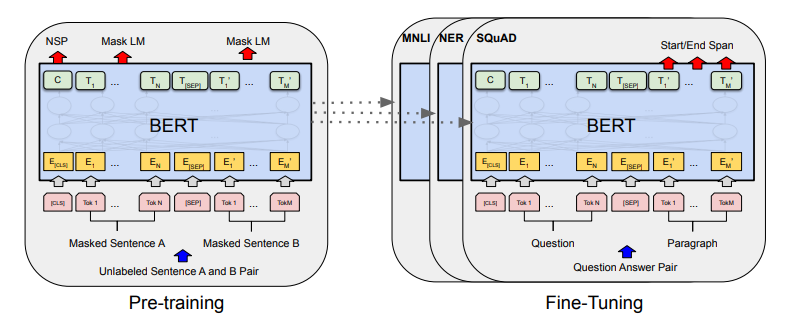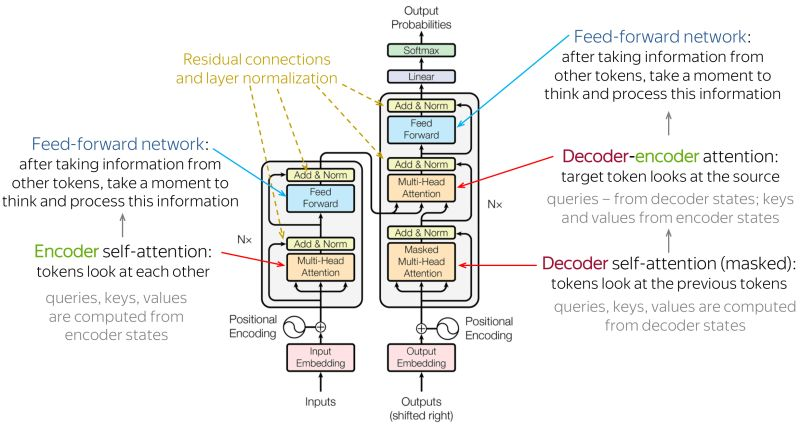## InfinityCodeX

Check out our blogs where we cover topics such as Python, Data Science, Machine Learning, Deep Learning. A Best place to start your AI career for beginner, intermediate peoples.

#BERT

BERT, which stands for Bidirectional Encoder Representations from Transformers, is a deep learning-based language model developed by Google that has been making waves in the field of natural language processing (NLP). BERT is unique in its ability to understand the context of words in a sentence and make predictions about the likelihood of a word being in a particular context. This has made BERT a highly versatile and powerful tool for solving a wide range of NLP tasks, such as sentiment analysis, question answering, and text classification.

## BERT's Architecture

BERT's architecture is based on the transformer network, which was introduced in 2017 by Vaswani et al. The transformer network is a neural network architecture designed specifically for NLP tasks and has since been used in many state-of-the-art NLP models.

BERT's architecture consists of two main components: an encoder and a decoder. The encoder is responsible for encoding the input text data into a hidden representation, while the decoder is responsible for predicting the likelihood of a word being in a particular context based on the encoded representation.

The input to BERT is a sequence of tokens, where each token represents a word in the sentence. The tokens are fed into the encoder, which is composed of multiple layers of attention mechanisms and feed-forward networks. The attention mechanism allows the model to focus on specific parts of the input sequence and weigh their importance in determining the encoded representation.

The encoded representation is then fed into the decoder, which is composed of a single feed-forward network. The decoder outputs a probability distribution over the vocabulary for each token in the input sequence. The decoder's predictions are then compared to the actual labels (i.e., the words in the sentence) to calculate the loss, which is used to update the model's parameters during training.

## BERT's Unique Features

BERT is unique among NLP models in several ways. First, BERT is bidirectional, meaning that it considers both the left and right context of each word in the sentence. This allows BERT to capture the context of words more accurately and outperforms unidirectional models.

Second, BERT is pre-trained on a massive amount of text data, which allows it to perform well on a wide range of NLP tasks without the need for task-specific training data. This makes BERT highly versatile and cost-effective, as it can be fine-tuned for specific NLP tasks with relatively small amounts of task-specific data.

Finally, BERT uses masked language modeling as its pre-training task, where a percentage of the tokens in the input sequence is masked and the model is trained to predict the masked tokens. This pre-training task helps BERT capture the context of words in a sentence more effectively and outperforms models trained with traditional pre-training tasks.

## BERT in Action

BERT has been applied to a wide range of NLP tasks and has consistently outperformed previous state-of-the-art models. For example, in the GLUE benchmark, which evaluates the performance of NLP models on a range of benchmark datasets, BERT outperformed previous state-of-the-art models by a substantial margin.

BERT has also been used to solve more complex NLP tasks, such as question answering and text summarization. For example, BERT has been fine-tuned to answer questions based on a large corpus of text data, such as the SQuAD dataset. BERT has also been used to generate summaries of long pieces of text, such as news articles.

# Transformers in BERT: Technical Math and Steps to Create the ModelTransformer

The transformer architecture is at the heart of the BERT model and is responsible for its impressive performance in natural language processing (NLP) tasks. In this section, we will provide a detailed explanation of the math behind transformers and the steps involved in creating a BERT model.

The Transformer architecture is a deep neural network that is used for sequence processing tasks such as language translation and text generation. The key idea behind the Transformer architecture is the use of self-attention mechanisms to dynamically weight the importance of different inputs in a sequence.

A Transformer consists of the following components:

2. Pointwise feedforward network

3. Residual connections and layer normalization

The following equations explain each component in detail:

1. Multi-head attention mechanism: The multi-head attention mechanism calculates attention scores between a query matrix Q, a key matrix K, and a value matrix V. The attention scores are calculated using the following equation:

Attention(Q,K,V) = softmax(QK/√d_k)V

Where d_k is the dimensionality of the key vectors and softmax is applied row-wise to the scores. The attention scores are then used to weigh the importance of the values.

2. Pointwise feedforward network: The Pointwise feedforward network is a simple two-layer feedforward neural network that is applied to each position in the sequence independently. The following equation represents the pointwise feedforward network:

FFN(x) = max(0,xW1 + b1)W2 + b2

Where W1 and W2 are the weights of the two layers and b1 and b2 are the biases. The activation function used is the ReLU activation.

3. Residual connections and layer normalization: Residual connections are used to add the input to the output of each layer to improve the flow of information through the network. The following equation represents the residual connection:

Output = Layer_Output + Input

Layer normalization is used to normalize the activations of each layer, which helps to stabilize the training process and improve the performance of the network. The following equation represents the layer normalization:

LayerNorm(x) = (x - mean(x)) / variance(x)

These components of the Transformer architecture are combined to form the final network, where the multi-head attention mechanism and pointwise feedforward network are applied alternately, followed by residual connections and layer normalization. The resulting network is capable of processing sequences in parallel, which greatly reduces the training time compared to traditional RNN-based models.

## Self-Attention Mechanisms

Self-attention mechanisms are the key component of transformers and allow the model to weight the importance of different parts of the input data. This is done by computing attention scores, which represent the importance of each input element relative to all other elements.

The attention scores are computed using a set of learnable parameters and are used to compute a weighted sum of the input elements. This weighted sum is then passed through a feedforward neural network to produce the final representation of the input data.

Mathematically, the attention mechanism can be represented as follows:

$$Attention(Q,K,V) = softmax(\frac{QK^T}{\sqrt{d_k}})V$$

Where Q, K and V are the query, key and value matrices, respectively, and $d_k$ is the dimension of the keys.

## Creating a BERT Model

The steps involved in creating a BERT model are as follows:

1.) Pre-processing: BERT requires the input data to be pre-processed and tokenized into subwords. This is done using a tokenizer that splits words into subwords and adds special tokens to represent the start and end of sentences.

2.) Encoding: The input data is then passed through a series of transformer layers to produce an encoded representation. Each transformer layer consists of a self-attention mechanism and a feedforward neural network.

3.) Fine-tuning: The pre-trained BERT model can be fine-tuned on a specific NLP task, such as sentiment analysis or question answering, by adding task-specific layers on top of the encoded representation.

4.) Training: The fine-tuned model is then trained on a labeled dataset to adjust the parameters of the self-attention mechanism and feedforward neural network.

5.) Evaluation: Finally, the model is evaluated on a held-out dataset to measure its performance on the task.

In conclusion, the use of transformers and self-attention mechanisms in BERT has revolutionized NLP by allowing models to understand the relationships between words and sentence structure in a way that was not possible with traditional models. The combination of pre-training and fine-tuning has made it possible to create models that achieve state-of-the-art performance on a wide range of NLP tasks.

## BERT Model Simple Sentiment Analysis Example



# pip install ktrain

# git clone https://github.com/laxmimerit/IMDB-Movie-Reviews-Large-Dataset-50k.git

import tensorflow as tf
import pandas as pd
import numpy as np
import ktrain
from ktrain import text
import tensorflow as tf

data_train = pd.read_excel('IMDB-Movie-Reviews-Large-Dataset-50k/train.xlsx', dtype = str)
data_test = pd.read_excel('IMDB-Movie-Reviews-Large-Dataset-50k/test.xlsx', dtype = str)

(x_train,y_train),(x_test,y_test),preprocess=text.texts_from_df(data_train,text_column='Reviews'
,label_columns='Sentiment',val_df=data_test
,maxlen=400,preprocess_mode='bert')

model = text.text_classifier(name='bert',train_data=(x_train,y_train)
,preproc=preprocess)

learner = ktrain.get_learner(model=model, train_data=(x_train, y_train),
val_data = (x_test, y_test),
batch_size = 6)

learner.fit_onecycle(lr = 2e-5, epochs = 1)

predictor = ktrain.get_predictor(learner.model, preprocess)
predictor.save('/content/drive/My Drive/bert')

data = ['this movie was horrible, the plot was really boring. acting was okay',
'the fild is really sucked. there is not plot and acting was bad',
'what a beautiful movie. great plot. acting was good. will see it again']

predictor.predict(data)

>>> ['neg', 'neg', 'pos']



So we hope that you enjoyed this project. If you did then please share it with your friends and spread this knowledge.